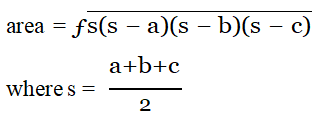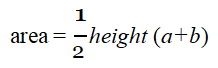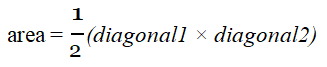Java Programs

Program 

Design a program to accept a day number (between 1 and 366), year (in 4 digits) from the user to generate and display the corresponding date. Also, accept ‘N’ (1 <= N <= 100) from the user to compute and display the future date corresponding to ‘N’ days after the generated date. Display an error message if the value of the day number, year and N are not within the limit or not according to the condition specified.

Test your program with the following data and some random data:

Example 1

INPUT:

DAY NUMBER: 255

YEAR: 2018

DATE AFTER (N DAYS): 22

OUTPUT:

DATE: 12 TH SEPTEMBER, 2018

DATE AFTER 22 DAYS: 4 TH OCTOBER, 2018

Example 2

INPUT:

DAY NUMBER: 360

YEAR: 2018

DATE AFTER (N DAYS): 45

OUTPUT:

DATE: 26 TH DECEMBER, 2018

DATE AFTER 45 DAYS: 9 TH FEBRUARY, 2019

Example 3

INPUT:

DAY NUMBER: 500

YEAR: 2018

DATE AFTER (N DAYS): 33

OUTPUT:

DAY NUMBER OUT OF RANGE.

Example 4

INPUT:

DAY NUMBER: 150

YEAR: 2018

DATE AFTER (N DAYS): 330

OUTPUT:

DATE AFTER (N DAYS) OUT OF RANGE.

Program 

A Prime-Adam integer is a positive integer (without leading zeros) which is a Prime as well as an Adam number.

Prime Number : A number which has only two factors, i.e. 1 and the number itself.

Example: 2, 3, 5, 7 …etc.

Adam number: The square of a number and the square of its reverse are reverse to each other.

Example : If n=13 and reverse of ‘n’ =31, then,

(13)2  = 169

(31)2  = 961 which is reverse of 169

thus 13, is an Adam number.

Accept two positive integers m and n, where m is less than n as user input. Display all Prime-Adam integers that are in the range between m and n (both inclusive) and output them along with the frequency, in the format given below:

Test your program with the following data and some random data:

Example 1

INPUT:

m=5

n=100

OUTPUT:

11 13 31

FREQUENCY OF PRIME-ADAM INTEGERS IS: 3

Example 2

INPUT:

m=100

n=200

OUTPUT:

101 103 113

FREQUENCY OF PRIME-ADAM INTEGERS IS: 3

Example 3

INPUT:

m=50

n=70

OUTPUT:

NIL

FREQUENCY OF PRIME-ADAM INTEGERS IS: 0

Example 4

INPUT:

m=700

n=450

OUTPUT: INVALID INPUT.

Program 

Write a program to print all the Krishnamurti number from 1 to n. Here, n is user dependent. A Krishnamurti number is a number whose sum of factorial of individual digits equals the number.

For example, 145 = 1! + 4! + 5! = 1 + 24+ 120 = 145.

Program 

Design a class to overload a function compare() as follows:

(a) void compare(int,int) - to compare tow integer values and print the greater of the tow integers.

(b) void compare(char,char) - to compare the numeric value of tow character with higher numeric value.

(c) void compare(String,String) - to compare the length of the tow strings and print the longer of the two.

Program 

Design a class to overload a function polygon() as follows:

(i) void polygon(int n, char ch) - with one integer argument and one character type argument that draws a filled square of side n using the character stored in ch.

(ii) void polygon(int x, int y) - with two integer arguments that draws a filled rectangle of length x and breadth y, using the symbol ‘@’

(iii) void polygon() - with no argument that draws a filled triangle shown below.

Sample I/O

(i) Input value of n = 2, ch=’O’

Output:

OO

OO

(ii) Input value of x = 2, y = 5

Output:

@@@@@

@@@@@

(iii) Output:

*

**

***

Program 

The International Standard Book Number (ISBN) is a unique numeric book identifier which is printed on every book. The ISBN is based upon a 10-digit code. The ISBN is legal if:

1 x digit1 + 2 x digit2 + 3 x digit3 + 4 x digit4  + 5 x digit5  + 6 x digit6  + 7 x digit7 + 8 x digit8  + 9 x digit9 + 10 x digit10 is divisible by 11.

Example:

For an ISBN 1401601499

Sum=1 x 1 + 2 x 4 + 3 x 0 + 4 x 1 + 5 x 6 + 6 x 0 + 7 x 1 + 8 x 4 + 9 x 9 + 10 x 9 = 253 which is divisible by 11.

Write a program to:

(i) Input the ISBN code as a 10-digit integer.

(ii) If the ISBN is not a 10-digit integer, output the message, "Illegal ISBN" and terminate the program.

(iii) If the number is 10-digit, extract the digits of the number and compute the sum as explained above. If the sum is divisible by 11, output the message, "Legal ISBN". If the sum is not divisible by 11, output the message, "Illegal ISBN".

Program 

Design a class to overload a function area() as follows:

1. double area(double a, double b, double c) with three double arguments, returns the area of a scalene triangle using the formula:2. double area(int a, int b, int height) with three integer arguments, returns the area of a trapezium using the formula:3. double area(double diagonal1, double diagonal2) with two double arguments, returns the area of a rhombus using the formula:Program 

A positive whole number ‘n’ that has ‘d’ number of digits is squared and split into two pieces, a right-hand piece that has ‘d’ digits and a left-hand piece that has remaining ‘d’ or ‘d-1’ digits. If the sum of the two pieces is equal to the number, then ‘n’ is a Kaprekar number. The first few Kaprekar numbers are: 9, 45, 297 ……..

Example 1:

9

92 = 81, right-hand piece of 81 = 1 and left hand piece of 81 = 8 Sum = 1 + 8 = 9, i.e. equal to the number.

Example 2:

45

452 = 2025, right-hand piece of 2025 = 25 and left hand piece of 2025 = 20 Sum = 25 + 20 = 45, i.e. equal to the number.

Example 3:

297

2972 = 88209, right-hand piece of 88209 = 209 and left hand piece of 88209 = 88 Sum = 209 + 88 = 297, i.e. equal to the number.

Given the two positive integers p and q, where p < q, write a program to determine how many Kaprekar numbers are there in the range between p and q (both inclusive) and output them.

The input contains two positive integers p and q. Assume p < 5000 and q < 5000. You are to output the number of Kaprekar numbers in the specified range along with their values in the format specified below:

SAMPLE DATA:

INPUT:

p = 1

q = 1000 OUTPUT:

THE KAPREKAR NUMBERS ARE:- 1, 9, 45, 55, 99, 297, 703, 999

FREQUENCY OF KAPREKAR NUMBERS IS: 8

Program 

A prime palindrome integer is a positive  integer  (without  leading  zeros)  which  is  prime  as  well as a palindrome. Given two positive integers m and n, where m < n, write a program to determine how many  prime-palindrome integers  are  there  in  the  range  between  m  and  n  (both inclusive) and output them.

The input contains two positive integers  m  and  n  where  m  <  3000  and  n  <  3000.  Display  the number of prime-palindrome integers in the specified range along with their values in the format specified below:

Test your program with the sample data and some random data:

Example 1

INPUT: m = 100 n = 1000

OUTPUT: THE PRIME PALINDROME INTEGERS ARE:

101, 131, 151, 181, 191, 313, 353, 373, 383, 727, 757, 787, 797, 919, 929

FREQUENCY OF PRIME PALINDROME INTEGERS : 15

Example 2

INPUT: m = 100 n = 5000

OUTPUT: OUT OF RANGE

Program 

A Composite Magic number is a positive integer which is composite as well as a magic number. Composite number: A composite number is a number that has more than two factors.

For example: 10

Factors are: 1, 2, 5, 10

Magic number: A magic number is a number in which the eventual sum of the digits is equal to 1

For example: 28=2+8=10=1+0=1

Accept two positive integers m and n, where m is less than n as user input. Display the number of Composite magic integers that are in the range between m and n (both inclusive) and output them along with the frequency, in the format specified below.

Test your program with the sample data and some random data:

Example 1:

INPUT: m = 10 n = 100

OUTPUT:

THE COMPOSITE MAGIC INTEGERS ARE: 10, 28, 46, 55, 64, 82, 91, 100

FREQUENCY OF COMPOSITE MAGIC INTEGERS IS: 8

Example 2:

INPUT: m = 1200 n = 1300

OUTPUT:

THE COMPOSITE MAGIC INTEGERS ARE:

1207, 1216, 1225, 1234, 1243, 1252, 1261, 1270, 1288

FREQUENCY OF COMPOSITE MAGIC INTEGERS IS: 9

Example 3:

INPUT: m = 120 n = 99

OUTPUT:

INVALID INPUT

Program 

A Goldbach number is a positive even integer that can be expressed as the sum of two odd primes. Note: All even integer numbers greater than 4 are Goldbach numbers. Example: 6 = 3 + 3, 10 = 3 + 7, 10 = 5 + 5 Hence, 6 has one odd prime pair 3 and 3. Similarly, 10 has two odd prime pairs, i.e. 3 and 7, 5 and 5.

Write a program to accept an even integer ‘N’ where N > 9 and N < 50. Find all the odd prime pairs whose sum is equal to the number ‘N’.

Test your program with the following data and some random data:

Example 1: INPUT: N = 14

OUTPUT: PRIME PAIRS ARE: 3, 11 7, 7

Example 2: INPUT: N = 30

OUTPUT: PRIME PAIRS ARE: 7, 23 11, 19 13, 17

Example 3: INPUT: N = 17

OUTPUT: INVALID INPUT. NUMBER IS ODD.

Example 4: INPUT: N = 126

OUTPUT: INVALID INPUT. NUMBER OUT OF RANGE.

Program 

Write java Function to check number is prime or not using function